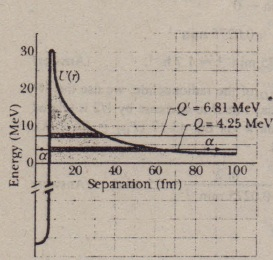# Alpha Decay

Alpha Decay

When a nucleus undergoes alpha decay, it transforms to a ‘different nuclide by emitting an alpha particle (a helium nucleus, 4He). For example, when uranium 238U undergoes alpha decay, it transforms to thorium

This alpha decay of 238U can occur spontaneously (without an external source of energy) because the total mass of the  decay products 234Th and 4He is less than  the mass of the original 238U. Thus, the total mass energy of the decay products is  less than the mass energy of the original nuclide. As defined by Eq. 38-47, in such a process the difference between the initial mass energy and the total final mass 6.81 MeV· energy is called the Q of the process the difference in mass energy is the decay’s disintegration energy Q. The Q for the decay in Eq. 43-21 is 4.25 MeV-that
b o~ 20 40 60, 80 100 amount of energy is said to be released by the alpha decay of ~38U, with the energy Separation 1fm) – transferred from mass energy to the kinetic energy  of the two products. The half-life of 238U for this decay process is 4.5 x 109 y. Why so long? If can decay in this way, why doesn’t every 3MNuclides in a sample of  atoms simply decay at once? To answer the questions. we must examine the process of alpha decay.
We choose a model in which the alpha panicle is imagined to exist (already formed) inside the nucleus before it escapes from the nucleus. Figure 43-9 shows the approximate potential energy V(r) of the system consisting of the alpha panicle and the residual 234Th nucleus, as a function of their separation r, This energy is a  combination of (I) the potential energy associated with the (attractive) strong nuclear force that acts in the nuclear interior and (2) a Coulomb potential associated with the (repulsive) electric force that acts between the two particles before and after the decay has occurred.  The horizontal black line marked Q = 4.25 MeV shows the disintegration energy for the process. If we assume that this represents the total energy of the alpha particle during the decay process, then the part of the VCr curve above this line constitutes a potential energy barrier like that in . This barrier cannot be  surmounted. If the alpha particle were able to be at some separation r within the barrier, its potential energy V would exceed its total energy E. This would mean, classically, that its kinetic energy K (which equals E – V) would be negative, an  impossible situation.(You should verify that both nuclear charge and the number of nucleons are conserved in this process.) Using the : Key Idea as  in pan (a) and proceeding as we did there. we would find that the mass of the two decay products (= 237.051 21 u + 1.00783 u)would exceed the mass of 2JRU by !1m = 0.008 25 u, with disintegration energy Q = -7,68 MeV. The minus sign indicates that we must add 7.68 MeV to a 2JAU nucleus before it will emit a proton; it will certainly not do so spontaneously.